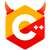# 3288. 成绩计算#include <cstdint>
#include <iostream>
#include <limits>
using namespace std;

int main() {
uint32_t t; cin >> t;
for (uint32_t query = 0; query < t; ++query) {
// Safely assume that there is at least one student.
int32_t crnt_max, crnt_min, crnt_sum;
cin >> crnt_sum; crnt_max = crnt_min = crnt_sum;
uint32_t cnt = 1;
for (int32_t score; cin >> score, score != -1; ++cnt) {
crnt_sum += score;
if (crnt_max < score)
crnt_max = score;
else if (crnt_min > score)
crnt_min = score;
}
cout << "case #" << query << ":\n" << crnt_max << '\n' << crnt_min << '\n' << crnt_sum / cnt << '\n';
}
}